International
Tables for
Crystallography
Volume B
Reciprocal space
Edited by U. Shmueli

International Tables for Crystallography (2010). Vol. B, ch. 1.4, p. 120   | 1 | 2 |

## Section 1.4.4.3. Effect of direct-space transformations

U. Shmuelia

#### 1.4.4.3. Effect of direct-space transformations

| top | pdf |

The phase shifts given in Table A1.4.4.1depend on the translation parts of the space-group operations and these translations are determined, all or in part, by the choice of the space-group origin. It is a fairly easy matter to find the phase shifts that correspond to a given shift of the space-group origin in direct space, directly from Table A1.4.4.1. Moreover, it is also possible to modify the table entries so that a more general transformation, including a change of crystal axes as well as a shift of the space-group origin, can be directly accounted for. We employ here the frequently used concise notation due to Seitz (1935)(see also IT A, 1983).

Let the direct-space transformation be given bywhere T is a nonsingularmatrix describing the change of the coordinate system and v is an origin-shift vector. The components of T and v are referred to the old system, andis the position vector of a point in the crystal, referred to the new (old) system, respectively. If we denote a space-group operation referred to the new and old systems byand, respectively, we haveIt follows from (1.4.4.2)and (1.4.4.5)that if the old entry of Table A1.4.4.1is given by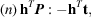the transformed entry becomes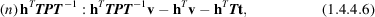and in the important special cases of a pure change of settingor a pure shift of the space-group origin (T is the unit matrix I), (1.4.4.6)reduces to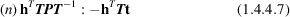or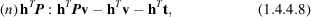respectively. The rotation matrices P are readily obtained by visual or programmed inspection of the old entries: if, for example,is khl, we must have,and, the remaining's being equal to zero. Similarly, ifis kil, where, we haveThe rotation matrices can also be obtained by reference to Part 7and Tables 11.2.2.1and 11.2.2.2in Volume A (IT A, 2005).

As an example, consider the phase shifts corresponding to the operation No. (16) of the space group(No. 129) in its two origins given in Volume A (IT A, 1983). For an Origin 2-to-Origin 1 transformation we find there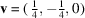and the old Origin 2 entry in Table A1.4.4.1is (16) khl (t is zero). The appropriate entry for the Origin 1 description of this operation should therefore be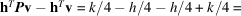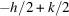, as given by (1.4.4.8), orif a trivial shift of 2π is subtracted. The (new) Origin 1 entry thus becomes: (16) khl:, as listed in Table A1.4.4.1.

### References

International Tables for Crystallography (1983). Vol. A, Space-Group Symmetry, edited by Th. Hahn. Dordrecht: Reidel.
International Tables for Crystallography (2005). Vol. A, Space-Group Symmetry, edited by Th. Hahn, 5th ed., corrected reprint. Heidelberg: Springer.
Seitz, F. (1935). A matrix-algebraic development of crystallographic groups. III. Z. Kristallogr. 90, 289–313.